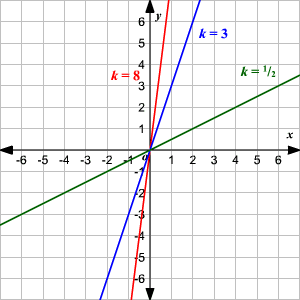# Direct Variation

Direct variation describes a simple relationship between two variables . We say $y$ varies directly with $x$ (or as $x$ , in some textbooks) if:

$y=kx$

for some constant $k$ , called the constant of variation or constant of proportionality . (Some textbooks describe direct variation by saying " $y$ varies directly as $x$ ", " $y$ varies proportionally as $x$ ", or " $y$ is directly proportional to $x$ .")

This means that as $x$ increases, $y$ increases and as $x$ decreases, $y$ decreases—and that the ratio between them always stays the same.

The graph of the direct variation equation is a straight line through the origin.Direct Variation Equation for $3$ different values of $k$

Example 1:

Given that $y$ varies directly as $x$ , with a constant of variation $k=\frac{1}{3}$ , find $y$ when $x=12$ .

Write the direct variation equation.

$y=\frac{1}{3}x$

Substitute the given $x$ value.

$\begin{array}{l}y=\frac{1}{3}\cdot 12\\ y=4\end{array}$

Example 2:

Given that $y$ varies directly as $x$ , find the constant of variation if $y=24$ and $x=3$ .

Write the direct variation equation.

$y=kx$

Substitute the given $x$ and $y$ values, and solve for $k$ .

$\begin{array}{l}24=k\cdot 3\\ \text{\hspace{0.17em}}\text{\hspace{0.17em}}k=8\end{array}$

Example 3:

Suppose $y$ varies directly as $x$ , and $y=30$ when $x=6$ . What is the value of $y$ when $x=100$ ?

Write the direct variation equation.

$y=kx$

Substitute the given $x$ and $y$ values, and solve for $k$ .

$\begin{array}{l}30=k\cdot 6\\ \text{\hspace{0.17em}}\text{\hspace{0.17em}}k=5\end{array}$

The equation is $y=5x$ . Now substitute $x=100$ and find $y$ .

$\begin{array}{l}y=5\cdot 100\\ y=500\end{array}$